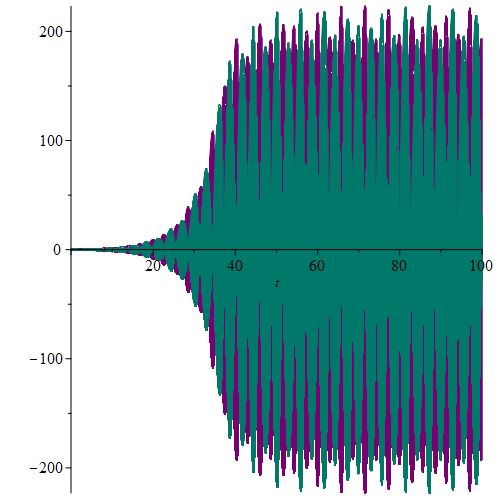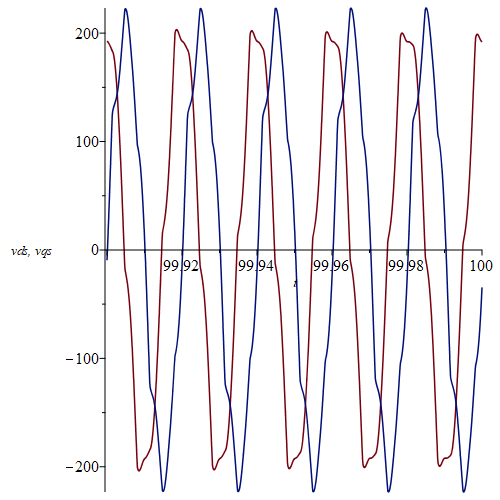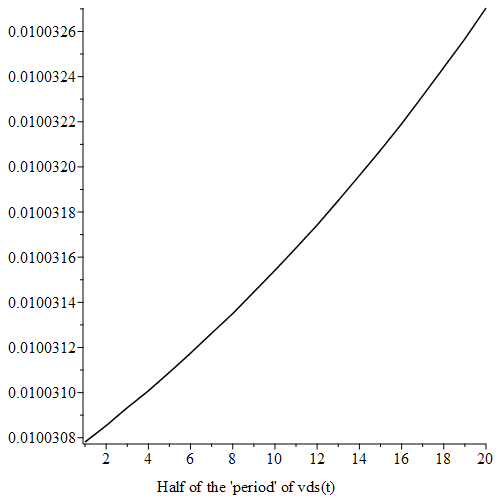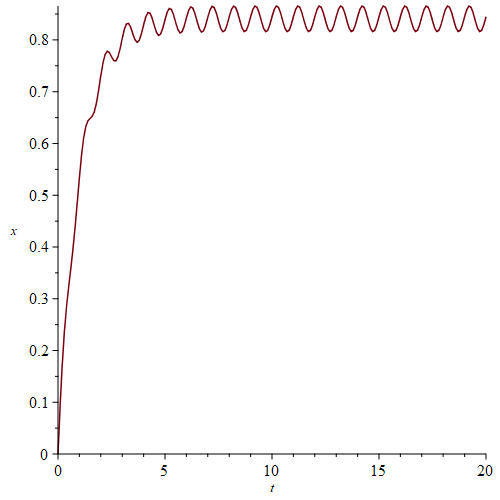## 22 Badges

16 years, 195 days

## An improved version...

@Alger As suggested by Dr. Venkat Subramanian I have added implicit=true and optimize=true.

Besides that I have made a procedure that on input of the value of C and the range of t-values computes the solution.

This is convenient when different C's are considered.

Computing times are much improved. A "singularity" is still announced for C = 0.000045 and range 0..100, but this time it doesn't happen till t = 81.598332, which again makes me distrust the existence of a singularity.

MaplePrimes20-04-30_odesystemIII.mw

Using method=ck45 (i.e. Cash-Karp) instead of the default rkf45 we don't get any complaints for C=0.000045 and range = 0..100:
With the procedure res from the uploaded worksheet do:

```VARS:=[[t,ids(t)],[t,ipdr(t)],[t,ipqr(t)],[t,iqs(t)],[t,vds(t)],[t,vqs(t)]];
dsol_45:=res(0.000045,0..100,method=ck45);
odeplot(dsol_45,VARS,0..100,numpoints=10000);
odeplot(dsol_45,VARS[-2..-1],99.9..100,numpoints=1000);
```

The plots:#################################
Added May 3.

Here is a slightly revised procedure res (called res2) which doesn't require the range argument, but allows it. The intention is to find the approximate period of a potentially periodic solution.

```#Allowing for leaving out the range simply by letting it not be a required parameter.
#It can be added in the form range= t0..t1 in the call to res2 if desired.
res2:= proc(c::realcons) global C;
dsolve(eval({eq1, eq2, eq3, eq4, ep5, ep6, ics},C=c), numeric,
maxfun = 10^8,compile=true,implicit=true,optimize=true,_rest)
end proc;
#Here the intention is to find the approximate period of a potentially periodic solution. We use #zeros of vds.
#The distance between two consequtive zeros times 2 should be the period in this case.
#We only take note of zeros beyond t=99.8 and up to 100.
dsol_45E2:=res2(0.000045,method=ck45,events=[[[vds(t),t>99.8],none]]);
dsol_45E2(99.805); # This first call takes a while
T1:=op(dsol_45E2(eventfired=)); # The event fired at this time
dsol_45E2(99.815); # This is fast because it starts from the result above.
T2:=op(dsol_45E2(eventfired=)); # The next zero
#Now automating this using the results T1 and T2:
A:=Array(datatype=float):
A(1):=T1:
A(2):=T2:
d:=T2-T1: eps:=1e-4: # eps = tolerance
for i from 3 while T2<=100 do
dsol_45E2(T2+d+eps);
T1:=T2;
T2:=op(dsol_45E2(eventfired=));
A(i):=T2;
d:=T2-T1
end do:

S:=[seq(A[i+1]-A[i],i=1..numelems(A)-1)]; # The consequtive differences between zeros
listplot(S);
wr; # The period of sin(wr*t) is 2*Pi/wr
2*Pi/wr;
```## odeplot...

Why not use odeplot instead of plot in the last plots too?

Here I take the code from acer's answer:

```CodeTools:-Usage(odeplot(dsol1,[t,(cos(wr*t)*vds(t) - sin(wr*t)*vqs(t))*sqrt(3)], 0 .. 40, labels=[t,'Uas'], color = ["Red"], style = [line],numpoints = 7500));
###
CodeTools:-Usage(odeplot(dsol1,[t,(cos(wr*t)*ids(t) - sin(wr*t)*iqs(t))], 0 .. 40, labels=[t,'Ias'], color = ["Red"], style = [line],numpoints = 7500));
```

## solve...

Using solve:

```restart;
eqs:=(-(2*I)*c1*eta+c1*sqrt(-e0^2-4*eta^2))/e0 = c2, ((2*I)*c2*eta+c2*sqrt(-e0^2-4*eta^2))/e0 = -c1;
solve({eqs},{c1,c2});
```

We see that c2 can be anything (c2=c2) and then if c2 is chosen, c1 is determined.

## Two variables only...

Go to ?pdsolve,numeric and you will find that the help page for pdsolve/numeric in the beginning in the section Parameters says:

PDEsys-single or set or list of time-dependent partial differential equations in two independent variables

Thus even if your equation had no integral pdsolve/numeric couldn't handle it.

## uneval modifier...

If I understand you correctly, what you could use is the uneval modifier in your procedure.

Here is the code for simplify2 with a few changes:

```restart;

simplify2 := proc (Term1::uneval)
# Simplify a term. Additionally check if the simplification is advantageous.
# In some cases the term gets longer after the simplification. Then discard.
# Give the local procedure variable a long name. They must not be identical in Term.
local simplify_tmpvar_c1, simplify_tmpvar_c1sum, simplify_tmpvar_c2, \
simplify_tmpvar_c2sum, simplify_tmpvar_nms, simplify_tmpvar_k, Term2,Term: #Term local now
# Assume all contents of the Term to be local variables to this procedure
# Otherwise, variables like "l1" can be overwritten by occurences in the calling worksheet.
Term:=eval(Term1,2); ############# change
simplify_tmpvar_nms:=convert(indets(Term,name),list): # get list of symbols
for simplify_tmpvar_k from 1 to LinearAlgebra:-ColumnDimension(simplify_tmpvar_nms) do
if simplify_tmpvar_nms[simplify_tmpvar_k] = Pi or simplify_tmpvar_nms[simplify_tmpvar_k] = 0 then
next: # 0 and Pi can not be variables
end if:
eval(sprintf("local %s;", String(simplify_tmpvar_nms[simplify_tmpvar_k]))); # assume local
end do:

# Get computational effort before simplification
simplify_tmpvar_c1:=add(codegen:-cost~(Term)):
simplify_tmpvar_c1sum := diff(simplify_tmpvar_c1,functions)+ \
diff(simplify_tmpvar_c1,additions)+ \
diff(simplify_tmpvar_c1,multiplications)+\
diff(simplify_tmpvar_c1,divisions):
# Perform simplification. Attention: tries to use global variables to simplify the expression (see above)
Term2 := simplify(Term):
# Get effort after simplification
simplify_tmpvar_c2:=add(cost~(Term2)):
simplify_tmpvar_c2sum := diff(simplify_tmpvar_c2,functions)+\
diff(simplify_tmpvar_c2,additions)+\
diff(simplify_tmpvar_c2,multiplications)+\
diff(simplify_tmpvar_c2,divisions):
if simplify_tmpvar_c2sum > simplify_tmpvar_c1sum then
# The simplification was not successful. Take old version.
Term2 := Term:
end if:
return Term2:
end proc:
#with(LinearAlgebra): with(ArrayTools): with(codegen): with(CodeGeneration): with(StringTools):

T := m2*(l1+l2)*(qD2)^2; # dummy-expression for kinetic energy
l1 := length(T);
T;
T_simpl := simplify2(T);

T_simpl;
l2 := length(T_simpl);
```

## A way...

```res:=[solve](1/2*m*v^2 = 1/2*F*x, v);
res1:=simplify(res) assuming positive;
combine(res1,radical) assuming positive;
```

## Stepwise...

Although I do think that the exact solver in this piecewise case should do this problem correctly, it can be done manually as I have here.
It should be noted that due to a bug in Maple 2020 this only works in previous versions (well at least in Maple 2019.2 and 2018.2, and also in the one you are using Maple 2015):

```restart;
with(plots):
Digits:=20:
perturbation := .9*(diff(epsilon(t), t, t)) = (-28.67085587*piecewise(0.2000000000e-1*t < 0.8e-1, 0., 0.2000000000e-1*t < .12, -0.8e-1+0.2000000000e-1*t, 0.2000000000e-1*t < .14, .16-0.2000000000e-1*t, 0.2e-1)-0.7645561571e-1*t-1.548363347)*(diff(epsilon(t), t))-0.7596363e-1*piecewise(0.2000000000e-1*t < 0.8e-1, 0., 0.2000000000e-1*t < .12, -0.8e-1+0.2000000000e-1*t, 0.2000000000e-1*t < .14, .16-0.2000000000e-1*t, 0.2e-1)-0.202569683e-3*t+0.26997403e-1-10.10*epsilon(t)
P:=simplify(perturbation,piecewise);
ivp:={P,epsilon(t0)=e0, D(epsilon)(t0)=e0_1}; # For general use
ivp0:=eval(ivp,{t0=0,e0=0,e0_1=0}) assuming t<4;
EPS1:=rhs(dsolve(ivp0));
e4,e4_1:=op((evalf@eval)~([EPS1,diff(EPS1,t)],t=4));
e4,e4_1:=op(simplify(fnormal([e4,e4_1]),zero));
ivp4:=eval(ivp,{t0=4,e0=e4,e0_1=e4_1}) assuming t>=4,t<6;
EPS2:=rhs(dsolve(ivp4));
plot(piecewise(t<4,EPS1,EPS2),t=0..6);
e6,e6_1:=op((evalf@eval)~([EPS2,diff(EPS2,t)],t=6));
e6,e6_1:=op(simplify(fnormal([e6,e6_1]),zero));
ivp6:=eval(ivp,{t0=6,e0=e6,e0_1=e6_1}) assuming t>=6,t<7;
EPS3:=rhs(dsolve(ivp6));
plot(piecewise(t<4,EPS1,t<6,EPS2,EPS3),t=0..7);
e7,e7_1:=op((evalf@eval)~([EPS3,diff(EPS3,t)],t=7)); # No small imaginary parts
ivp7:=eval(ivp,{t0=7,e0=e7,e0_1=e7_1}) assuming t>=7;
EPS4:=rhs(dsolve(ivp7));
EPS:=piecewise(t<4,EPS1,t<6,EPS2,t<7,EPS3,EPS4): # The solution
plot(EPS,t=0..10,color=blue); pexact:=%:
### Now numerically:
numsol := dsolve({perturbation, epsilon(0)=0, D(epsilon)(0)=0}, numeric,abserr=1e-20,relerr=1e-18,maxfun=0):

odeplot(numsol, [t, epsilon(t)], t=0..10, gridlines=true, color=red); pnumeric:=%:
display(pexact,pnumeric); # No difference discernible
odeplot(numsol,[t, epsilon(t)-EPS],0..10); # The difference
```

Note:  The problem in Maple 2020 shows up here:

EPS3:=rhs(dsolve(ivp6));
Error, (in dsolve) invalid left hand side of assignment

## frontend...

frontend does a good job here. Using the example by Tom Leslie (resembling yours):

```restart;
expr:=(diff(P(t),t)+sin(Pi*x/L)*a(t))/(L^4*k*A*G)+sin(Pi*x/L)*diff(a(t),t\$4)+sin(Pi*x/L)*diff(P(t),t);
####
frontend(coeff,[expr,a(t)]);
frontend(coeff,[expr,diff(P(t),t)]);
frontend(coeff,[expr,sin(Pi*x/L)]);
```

## The loop...

The "end do" in the loop should clearly be at the end.
So with that corrected your code runs:

```restart;
with(plots):
with(plottools):
K := 48;
R1 := 30; R2 := 20; OA := R1+R2;
Vodilo := PLOT3D(cuboid([-3, -3, 0], [OA+4, 4, 2]));
Koleso1 := PLOT3D(cylinder([0, 0, 5], R1, 18), cylinder([0, 0, -2], 1, 16));
Koleso2 := PLOT3D(cylinder([0, 0, 7], R2, 6), cylinder([0, 0, -2], 1, 16));
Amplituda := .3:
###
for i from 0 to K do
phi := sin(6.28*i/K)*Amplituda;
phi1 := phi*(R1+R2)/R2;
P1 := rotate(Koleso2, 0, 0, -phi1);
P2 := rotate(Vodilo, 0, 0, -phi);
x := OA*cos(phi);
y := OA*sin(phi);
P[i] := display(Koleso1, translate(P1, x, y, 0), P2)
end do: # colon
###

display(seq(P[i], i = 0 .. K), orientation = [55, 101], insequence = true, scaling = constrained, axes = normal);
```

## Maybe this...

This unassigns all the numbered members:

```restart;
with(Statistics):
X := a -> RandomVariable(Exponential(a)):
##
for n from 1 to 100 do
S := X(n);
# here are some operations to do
end do:
##
A:={anames(alluser)}:
IA:=indets(A,suffixed(_ProbabilityDistribution,integer)):
##
eval(_ProbabilityDistribution5);
exports(_ProbabilityDistribution5);
##
unassign(op(IA));
eval(_ProbabilityDistribution5);
```

But you might want to unassign all but the last one:

```N:=100:
for n from 1 to N do
S := X(n);
# here are some operations to do
if n = N-1 then
A:={anames(alluser)};
IA:=indets(A,suffixed(_ProbabilityDistribution,integer));
unassign(op(IA));
end if
end do:
anames(alluser);
```

## Infinitely many solutions...

Your integral equation has infinitely many solutions because the corresponding homogeneous equation has a one parameter family of solutions.
A particular solution can be found as vv did.

You will find my reasoning in the attached worksheet:

https://www.mapleprimes.com/questions/228874-Collocation-Method-For-Integral-Equation-#answer266560

Preben Alsholm, March 17 2020.
Additions made at the bottom March 18 2020.

 > restart;

Notice that I have replaced the coefficient 1/2 of the integral with the parameter a:

 > eq := Z(x) =  3/2-9/2*exp(-2*x)-9/2*exp(-x)+a*int(exp(-y)*Z(x-y), y= 0..ln(2));
 > EQ:=IntegrationTools:-Change(eq, x-y=t, t);

The corresponding homogeneous equation:

 > EQ0:=Z(x) = a*Int(exp(t - x)*Z(t), t = x - ln(2) .. x);

We find a particular solution to the inhomogeneous equation following vv:

 > ZZ:=x -> A*exp(-2*x)+B*exp(-x)+C;
 > expand(eval( (lhs-rhs)(EQ), Z=ZZ));
 > coeffs(%, [exp(x),exp(2*x)]);
 > ABC:=solve([%],{A,B,C});
 > Zsol:=eval(ZZ(x),ABC);
 > simplify(eval( (lhs-rhs)(EQ), Z=unapply(Zsol,x))); # Check

Now find solutions of the homogeneous equation by first differetiating it and thereby getting a delay differential equation:

 > INT:=combine(-1*(rhs=lhs)(EQ0)); # For use below
 > dde01:=(combine@expand)(diff(EQ0,x));

The delay differential equation:

 > dde0:=eval(dde01,INT);

The equation dde0 has as one solution Z(x) = exp(-x) for all values of a:

 > eval((lhs-rhs)(dde0),Z=(x->exp(-x)));
 > simplify(%);

but notice that Z(x) = exp(-x)  doesn't satisfy  the homogeneous integral equation unless a = 1/ln(2):

 > eval((lhs-rhs)(EQ0),Z=(x->exp(-x)));
 > factor(value(%));

Trying to find other solutions to dde0 of the form Z(x) = exp(r*x):

 > value(eval((lhs-rhs)(dde0),Z=(x->exp(r*x))));
 > u:=(combine@expand)(%/exp(r*x)); # Needs to be zero
 > eval(u,r=-1);

Numerically we see here that for a < 0 there is one solution for r and for a > 0 two solutions except at (as it turns out) at a = 1/ln(2).

One of the solutions is -1.

 > plots:-animate(plot,[u,r=-8..2,-1..1,labels=[r,"u"]],a=-2..3,frames=100);
 > usol:=expand(solve(u=0,r,AllSolutions));
 > zz:=op(indets(usol,suffixed(_Z,integer)));

We take the two real branches:

 > rm1:=eval(usol,zz=-1);
 > r0:=eval(usol,zz=0);
 > plot([rm1,r0],a=-2..3,color=[red,"Green"],thickness=3,legend=["rm1","r0"]);

The two branches meet at a = 1/ln(2):

 > eval(rm1-r0,a=1/ln(2));

Checking that the solutions Z(x) = exp(rrm1*x) and  Z(x) = exp(r0*x) actually satisfy dde0:

First rm1:

 > rr:=expand(eval((lhs-rhs)(value(dde0)),Z=(x->exp(rm1*x))));
 > simplify(rr);
 > rr:=expand(eval((lhs-rhs)(value(dde0)),Z=(x->exp(r0*x))));
 > simplify(rr) ;

Now checking that they also satisfy EQ0, but remember that actually exp(-x) is not a solution unless a = 1/ln(2):

 > rr:=expand(eval((lhs-rhs)(value(EQ0)),Z=unapply(exp(rm1*x),x)));
 > simplify(rr); # I see!  It should only be zero for 0 < a <= 1/ln(2)

Testing with a = 1/2:

 > evalf(eval(rm1,a=1/2));
 > rrc:=expand(eval[recurse]((lhs-rhs)(value(EQ0)),{a=1/2,Z=unapply(exp(rm1*x),x)}));
 > simplify(%); # 0
 > evalf(rrc);  # 0.
 > rrcn:=normal(rrc);
 > simplify(denom(rrcn));
 > evalf(%); # -0.825...
 > simplify(numer(rrcn));

We look at r0.

 > rr:=expand(eval((lhs-rhs)(value(EQ0)),Z=unapply(exp(r0*x),x)));
 > simplify(rr); # # It should only be zero for 1/ln(2) <= a

We look at a = 1/2:

 > eval(r0,a=1/2);
 > evalf(%);
 > rrc:=expand(eval[recurse]((lhs-rhs)(value(EQ0)),{a=1/2,Z=unapply(exp(r0*x),x)}));
 > simplify(%); # 0
 > rrcn:=normal(rrc);
 > simplify(denom(rrcn));
 > evalf(%); # 0.
 > simplify(numer(rrcn)); # 0

The problem appears to be Maple's ability to show that some quantity is actually zero.

Let us do a similar concrete test of the case a = 3  ( > 1/ln(2) ).

We didn't choose a = 2 because it is rather special: rm1 = -1 and r0 = 0 are found without evalf.

 > evalf(1/ln(2));
 > eval(rm1,a=3);
 > evalf(%);
 > rrc:=expand(eval[recurse]((lhs-rhs)(value(EQ0)),{a=3,Z=unapply(exp(rm1*x),x)}));
 > evalf(rrc); # Float(undefined)* ...
 > rrcn:=normal(rrc);
 > simplify(denom(rrcn));
 > evalf(%); # 0.
 > simplify(numer(rrcn)); #0

We look at r0.

 > eval(r0,a=3);
 > evalf(%);
 > rrc:=expand(eval[recurse]((lhs-rhs)(value(EQ0)),{a=3,Z=unapply(exp(r0*x),x)}));
 > simplify(%); #0
 > rrcn:=normal(rrc);
 > simplify(denom(rrcn));
 > evalf(%); # 9.295 ...
 > simplify(numer(rrcn));

Thus we (believe we) have found the following one parameter family of solutions to the inhomogeneous integral equation EQ:

 > SOL:=Zsol+C*piecewise(a<=0,0,a<=1/ln(2),exp(rm1*x),exp(r0*x));

A direct check:

 > rr:=expand(eval((lhs-rhs)(value(EQ)),Z=unapply(SOL,x))):
 > simplify(%); #  0

An animation in C for a = 1/2 on x = 1.5 .. 3.5:

 > SOL1:=eval(SOL,a=1/2);
 > evalf(SOL1);
 > plots:-animate(plot,[SOL1,x=1.5..3.5],C=-2000..2000,trace=24,caption=typeset("a = ",1/2));

An animation in C for a = 3 and on x = -1 .. 2:

 > SOL2:=eval(SOL,a=3);
 > evalf(SOL2);
 > plots:-animate(plot,[SOL2,x=-1..2],C=-1..1,trace=24,caption="a = 3");
 > ###################################################################################################################

Added March 18 2020:

We can single out one of these solutions by imposing an initial condition.

We shall assume that a > 0 from now on, since otherwise the solution SOL has no C:

 > eval(SOL,x=0) assuming a>0;

If Z(0) = 0 is required then C is given by C = C0, where:

 > C0:=solve(%,C);

The solution Z(x) = SOL is then

 > SOL0:=eval(SOL,C=C0) assuming a>0;

If furthermore a = 1/2 the solution is

 > SOL12:=eval(SOL0,a=1/2);
 > plot(SOL12,x=0..10);
 > limit(SOL12,x=infinity);
 > Digits:=15:
 > eps:=1e-5: plots:-animate(plot,[SOL0,x=0..10],a=eps..1/ln(2)-eps);

To find the value of SOL0 for a = 1 we must take the limit:

 > SOL1:=limit(SOL0,a=1);
 > limit(SOL1,x=infinity); # 3
 > plot([SOL1,3],x=0..20,linestyle=[1,3],color=[red,black],caption="a = 1");

The value of SOL0 at 1/ln(2) is found by taking the limit:

 > SOL_ln:=limit(SOL0,a=1/ln(2));
 > L_ln:=limit(SOL_ln,x=infinity); # 3*ln(2)/(-1 + 2*ln(2))
 > evalf(L_ln);
 > plot([SOL_ln,L_ln],x=0..20,linestyle=[1,3],color=[red,black],caption=typeset("a = ",1/ln(2)));
 >
 >Download IntegralEquations_delay_Z.mw

## No way...

I have corrected some syntactical errors. But dsolve cannot handle this analytically.

Certainly numerically, though:

```restart;
ode:=m*diff(x(t),t,t)= - m * A * sin(2*Pi*f*t) - piecewise( abs(x(t)) < x__max, 0, abs(x(t)) >= x__max, -k*(abs(x(t))-x__max)*signum(x(t))) - diff(x(t), t);
dsolve(ode); #NULL
nms:=indets(ode,And(name,Not(constant))) minus {x,t};
res:=dsolve({ode,x(0)=x0,D(x)(0)=x1},numeric,parameters=[nms[],x0,x1]);
res(parameters=[A=1,m=1,f=1,x__max=2,k=2,x0=0,x1=1]); # Example
plots:-odeplot(res,[t,x(t)],0..20);
```## Only rank 2...

Try this:

```restart;
Rok := Vector([2013, 2014, 2015, 2016, 2017, 2018], datatype = float);

TrzbyCelkemEmco := Vector([1028155, 1134120, 1004758, 929584, 995716, 1152042], datatype = float);
########
KubickaTrzby := Statistics:-PolynomialFit(3, Rok, TrzbyCelkemEmco, x);
p1:=plot(KubickaTrzby,x=2013..2018):
p2:=plot(Rok,TrzbyCelkemEmco,color=blue):
plots:-display(p1,p2); # Not impressive
########
pol:=a*x^3+b*x^2+c*x+d;
resid:=eval~(pol,x=~Rok)-TrzbyCelkemEmco; # Residuals
A,B:=LinearAlgebra:-GenerateMatrix(convert(resid,list),[a,b,c,d]);
op(1,A); # Dimension of A: 6x4
LinearAlgebra:-Rank(A); # 2 only
```

The rank could in principle have been min(6,4) = 4. That would have meant full rank.

## A start...

To get an exact answer to this question is certainly not easy to say the least.

But the beginnings of an attempt is this:

```restart;
### Your right hand sides:
fS := -D2*x1*x2-D1*x1-x1*x3+S;
fV := D2*x1*x2-D4*x2+x1*x3;
fC := -D6*x3*x4-D5*x3+x2;
fR := D6*x3*x4-x4;
eqs:=solve({fS,fV,fC,fR},{x1,x2,x3,x4}); # Notice RootOfs
L:=map(subs,[eqs],[x1,x2,x3,x4]); # The equilibrium points
### The Jacobian matrix as a function of x1,x2,x3,x4:
J:=unapply(VectorCalculus:-Jacobian([fS,fV,fC,fR],[x1,x2,x3,x4]),x1,x2,x3,x4);
J(x1,x2,x3,x4); # View
J(op(L)); # Point number 1
EV1:=LinearAlgebra:-Eigenvalues(%);
### The first two are negative since D1>0.
### But the two other depend on the actual size of your parameters as seen here:
### For these values the system is asymptotically stable:
eval(EV1,{D1=0.1,D2=1,D4=0.1,D5=.1,S=.0001});
eval(EV1,{D1=0.1,D2=1,D4=0.1,D5=.1,S=.0001});
### For these values the system is unstable:
eval(EV1,{D1=0.1,D2=1,D4=0.1,D5=.1,S=1});
eval(EV1,{D1=0.1,D2=1,D4=0.1,D5=.1,S=1});
```

This just illustrated the problem for point 1.

## dsolve...

Without specifying Q concretely you get:

```restart;
ode:=diff(v(x),x,x)+diff(v(x),x)*cot(x)-v(x)*(cot(x)^2+nu)=a^2*Q(x)/D1;
dsolve(ode);
```

Notice that there is an unevaluated integral involved simply because Q is not known.

﻿